## Preliminaries

Make sure to move to the proper working directory and that the file NYC_Sub_borough_Area.dbf is in that directory.

Also, activate the tidyverse and foreign packages using the `library` command (the tidyverse package includes ggplot2):

``library(tidyverse)``
``````[30m── [1mAttaching packages[22m ────────────────────────── tidyverse 1.2.1 ──[39m
[30m[32m✔[30m [34mggplot2[30m 3.0.0     [32m✔[30m [34mpurrr  [30m 0.2.5
[32m✔[30m [34mtibble [30m 1.4.2     [32m✔[30m [34mdplyr  [30m 0.7.6
[32m✔[30m [34mtidyr  [30m 0.8.1     [32m✔[30m [34mstringr[30m 1.3.1
[32m✔[30m [34mreadr  [30m 1.1.1     [32m✔[30m [34mforcats[30m 0.3.0[39m
[30m── [1mConflicts[22m ───────────────────────────── tidyverse_conflicts() ──
``library(foreign)``

## A quick introduction to ggplot

We will be using the commands in the ggplot2 package for the descriptive statistics plots. There are many options to create nice looking graphs in R, including the functionality in base R, but we chose ggplot2 for its clean logic and its implementation of a grammar for graphics.1

An in-depth introduction to ggplot is beyond the current scope, but a quick overview can be found in the Data Visualization chapter of Wickham and Grolemund’s R for Data Science book, and full details are covered in Wickham’s ggplot2: elegant graphics for data analysis (2nd Edition) (Springer Verlag, 2016).

The logic behind ggplot is an implementation of Wilkinson’s grammar for graphics, using the concept of layers. These are the components that make up a plot, such as a data set, aesthetic mappings (variables for different aspects of the graph, such as the x and y-axes, colors, shapes, etc.), statistical transformations, a geometric object and position adjustments. Several layers can be drawn on top of each other, providing the ability to create incredibly complex graphs.

For now, the main parts to concentrate on are the data set and the aesthetics, or `aes`. The latter are typically (at least) the variables to be plotted. These are usually declared in the main ggplot command, e.g., `ggplot(dataset,aes(x=var1,y=var2))` and apply to all the following layers. However, they can also be specified for each layer individually.

Next follow one or more geometric objects, `geom_*` and various adjustments, added to the first command by means of a plus sign.

The terminology may seem a little unfamiliar at first, but as long as you remember that `aes` are the variables and the `geom_*` are the plot types, you will be on your way.

## Fundamentals - A Scatter Plot

The scatter plot shows the relationship between two variables as points with cartesian (x, y) coordinates matching the value for each variable, one on the x-axis, the other on the y-axis.

First, we use `read.dbf` from the foreign package to read the data from NYC_Sub_borough_Area.dbf into a data frame nyc.data.

``nyc.data <- read.dbf("NYC_Sub_borough_Area.dbf")``

Next, we turn this into a tibble (not absolutely necessary, but useful) using `as_tibble` and assign it back to the same object.

``nyc.data <- as_tibble(nyc.data)``

We can now print out the contents (there are 55 observations and 34 variables).

``nyc.data``

We check the variables using the `names` command.

``names(nyc.data)``
``````  "bor_subb"   "name"       "code"       "subborough"
 "forhis06"   "forhis07"   "forhis08"   "forhis09"
 "forwh06"    "forwh07"    "forwh08"    "forwh09"
 "hhsiz1990"  "hhsiz00"    "hhsiz02"    "hhsiz05"
 "hhsiz08"    "kids2000"   "kids2005"   "kids2006"
 "kids2007"   "kids2008"   "kids2009"   "rent2002"
 "rent2005"   "rent2008"   "rentpct02"  "rentpct05"
 "rentpct08"  "pubast90"   "pubast00"   "yrhom02"
 "yrhom05"    "yrhom08"   ``````

We will start by using the variables kids2000 (percentage households with children under 18 in 2000) and pubast00 (percentage households receiving public assistance in 2000). Before we move to the graphs, we check out the characteristics of their distribution using the familiar `summary` command. Remember, to pass a variable to `summary` you need to identify it with its data frame, either using the [[ ]] notation, or the \$ notation. Using the latter yields:

``summary(nyc.data\$kids2000)``
``````   Min. 1st Qu.  Median    Mean 3rd Qu.    Max.
8.382  30.301  38.228  36.040  42.773  55.367 ``````
``summary(nyc.data\$pubast00)``
``````   Min. 1st Qu.  Median    Mean 3rd Qu.    Max.
0.8981  3.3860  6.8781  8.4372 11.5369 23.4318 ``````

### Data - the data frame

The first aspect that needs to be set for any graph is which data frame contains the variables to be plotted. This is passed as the first argument to the `ggplot` command, as `ggplot(data=dataframe, ... )`, ignoring all other arguments for now (by itself, this command will not do anything). For simplicity sake, the argument `data` is often not mentioned explicitly, but for now we will spell out everything in full. In our example, the argument would be `data=nyc.data`.

### Aesthetics - the variables

The aesthetics, or `aes` are the basic visual ways in which values that correspond to given variables are represented in a two-dimensional graph (on the screen or on paper). These include the position according to the x and y axis, a size, a color, and a shape. All these elements can be associated with a variable, or set to a constant.

The aesthetics are set by passing the required arguments to the expression `aes( )` in the parentheses (it is easy to forget this initially). For example, for our scatter plot, we will set the x-axis to kids2000 and the y-axis to pubast00, as in `aes(x = kids2000,y = pubast00)`. Note that you don’t need to put parentheses around the variable names (that is a characteristics of all functions in the tidyverse, as we saw for the data frame manipulation).

For now, we will just use these two aspects of the graph. We will return to color, size and shape later.

### Geom - the type of graph

So far, we have just set up the main parameters for a graph. These are passed as arguments to the `ggplot` command. Think of them as the rules that apply to all the layers in the graph. The graph itself is constructed by adding different layers, each corresponding to a geometric object or to other aspects of the graph, such as labels, text annotations, styles, etc.

Each type of geometric object is given by a `geom_xxx` command. Each of these geom functions has built-in options to compute statistics, and, if needed, transformations, to be able to map the values in the variables to a geometric object in the graph.

For the scatter plot, the geometric object is a point, corresponding to the location for the x and y variable, represented by the `geom_point` geom.

### A basic scatter plot

We are now ready to construct our first graph, a bare bones scatter plot. In ggplot the different layers are added together by means of a + symbol. For the scatter plot, we have two elements: the main `ggplot` command that specifies the data frame and variables for the x and y axes (the `aes`); and the command to specify `geom_point`. Make sure to have the + sign at the end of the line (and not on the next line), and don’t forget the parentheses following `geom_point( )`. If all goes well, you will create the default scatter plot.

``````ggplot(data=nyc.data,aes(x = kids2000,y = pubast00)) +
geom_point()``````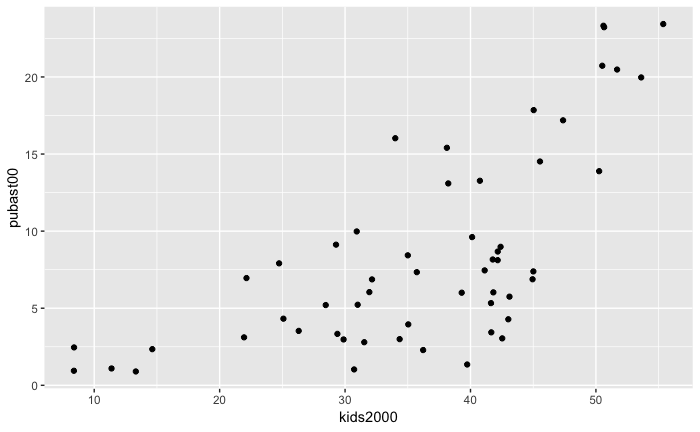## Color

While the scatter plot in essence depicts two variables, it is often useful to distinguish between groups of observations (in spatial lingo, we will call this spatial heterogeneity). There are several ways to implement this in ggplot, but first we will illustrate the use of `color` (we will consider `shape` and `size` next).

Color is an aesthetic. It can be set to a constant, or matched with a variable. In the case of a constant, we simply set it to an accepted color value, such as in `color = "red"`. Typically, it is specified as part of the `geom` to which it pertains.

### Color as a constant

Things can get a bit confusing, since the constant color can be specified as an option of the geom, without specifying the `aes`. For example, setting `geom_point(color="red")` will make all the points red.

``````ggplot(data=nyc.data,aes(x=kids2000,y=pubast00)) +
geom_point(color="red")``````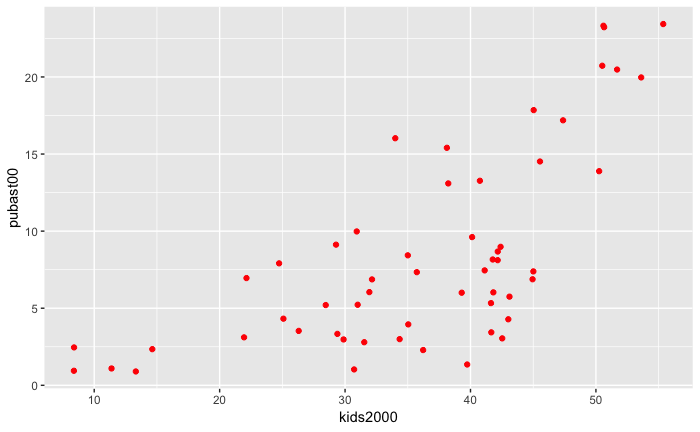Note the subtle difference in thinking with setting `aes(color="red")` for `geom_point`. The result is the same, but in essence now we don’t consider the color to be an option, but one of the aesthetics assigned to a constant value instead of to a variable. Remember to use the quotes around red. Otherwise, `red` is considered to be a variable, and since there is no such variable in our data frame, it will generate an error.

``````ggplot(data=nyc.data,aes(x = kids2000,y = pubast00)) +
geom_point(aes(color="red"))``````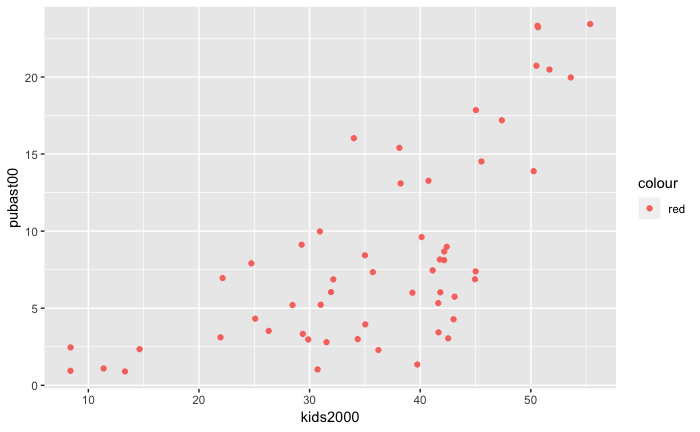Notice the difference in that now a legend is added with the value for the color (later, we will see how to customize the heading for the legend). This highlights how `color` is an aesthetic, in this case mapped to a constant value.

### Color as a variable

We can now use the same principle to tie the value of `color` to a variable instead of a constant. For example, say we want to distinguish between the points corresponding to sub-boroughs in the Bronx and Manhatten and those in the rest of the city. We assign the aesthetic `color` to the variable manbronx (note, no quotes since it is a variable name that is in the data frame).

``````ggplot(data=nyc.data,aes(x = kids2000, y = pubast00)) +
geom_point(aes(color=manbronx))``````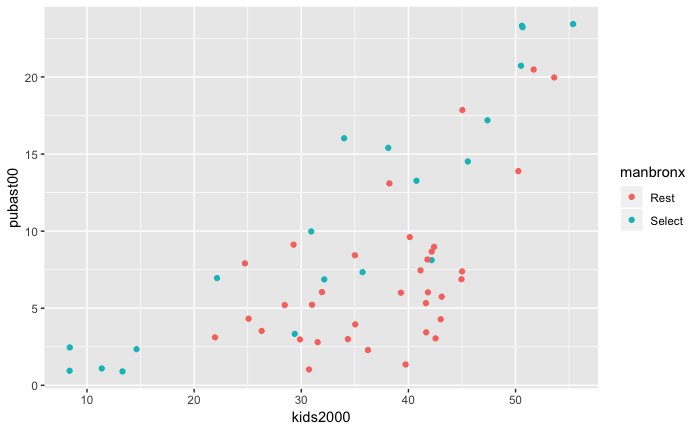Now, the legend heading is manbronx (the variable we specified) and the value for the two colors is given.

Alternatively, we could also have specified the `color` aesthetic in the main `ggplot` command, by means of `ggplot(data=nyc.data,aes(x = kids2000, y = pubast00, color=manbronx))`.

``````ggplot(data=nyc.data,aes(x = kids2000, y = pubast00, color=manbronx)) +
geom_point()``````The result is the same, but there is an important difference. By specifying the color aesthetic in the main command, we specify it for all following layers. In our simple example, there is only one layer, so it doesn’t really make a difference, but typically it makes more sense to specify aesthetics other than x and y specific to each geom. As we will see later, a graph is made up of several geoms, each potentially with their own aesthetics.

## Saving a Graph

### Writing a graph to a file

In our discussion so far, the graphs are drawn to the screen and then disappear. To save a ggplot graph to a file for publication, there are two ways to proceed. One is the classic R approach, in which first a device is opened, e.g., by means of a `pdf` command for a pdf file (each type of file has its own command). Next, the plot commands are entered, and finally the device is turned off by means of `dev.off()`.

Note that it is always a good idea to specify the dimension of the graph (in inches). If not, the results can be unexpected.

For example, to save our scatter plot in a pdf file scatter1.pdf with a height and width of 3 inches, we first set `pdf("scatter1.pdf",height=3,width=3)`. Next we enter our usual ggplot commands. Note that nothing will appear on the screen. Finally, we turn the “device” (i.e., the file) off by means of `dev.off()`.

``````pdf("scatter1.pdf",height=3,width=3)
g + geom_point(aes(color=manbronx))
dev.off()``````
``````null device
1 ``````

### Saving with `ggsave`

In addition to the standard R approach, ggplot also has the `ggsave` command, which does the same thing. It requires the name for the output file, but derives the proper format from the file extension. For example, an output file with a png file extension will create a png file, and similarly for pdf, etc.

The second argument specifies the plot. It is optional, and when not specified, the last plot is saved. Again, it is a good idea to specify the `width` and `height` (in inches). In addition, for raster files, the dots per inch (`dpi`) can be set as well. The default is 300, which is fine for most use cases, but for high resolution graphs, one can set the dpi to 600.

For example, we assign our scatter plot to scat1, and then save it using `ggsave("scatter2.png",scat1,height=3,width=3,dpi=600)`:

``````scat1 <- g + geom_point(aes(color=manbronx))
ggsave("scatter2.png",scat1,height=3,width=3,dpi=600)``````

The file will be added to the working directory.

To see the plot on your screen, simply type its name (scat1).

``scat1``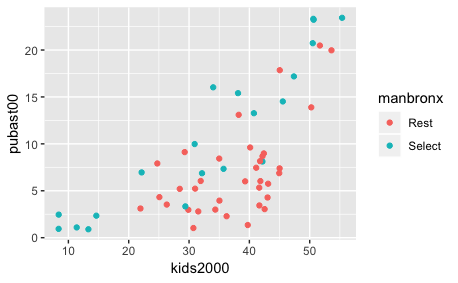### Saving an R object

Finally, yet another approach to keep a plot object is to assign the plot commands to a variable and then save this to disk, using the standard R command `saveRDS`. This can later be brought back into an R session using `readRDS`. To save the plot, we need to specify a file name with an .rds file extension, for example, scatter1.rds.

``saveRDS(scat1,"scatter1.rds")``

At some later point (or in a different R session), we can then read the object with `readRDS` and plot it. Note that we do not need to assign it to the same variable name as before. For example, here we call the graph object newplot.

``````newplot <- readRDS("scatter1.rds")
newplot``````## Shape

A further aesthetic is the shape of the geometric object. R has a number of pre-defined shapes with (to the novice) obscure codes, assigned by the `pch` command.2

Again, we can assign the aesthetic shape to either a constant (one of the codes) or to a variable.

### Setting the shape to a constant

Similar to how we set the color above, we can add an option `shape=17` to the `geom_point` command. This will generate a scatter plot with the points represented as triangles.

``g + geom_point(shape=12)``